Scalar product - Defination

Chapter 10 Class 12 Vector Algebra
Concept wise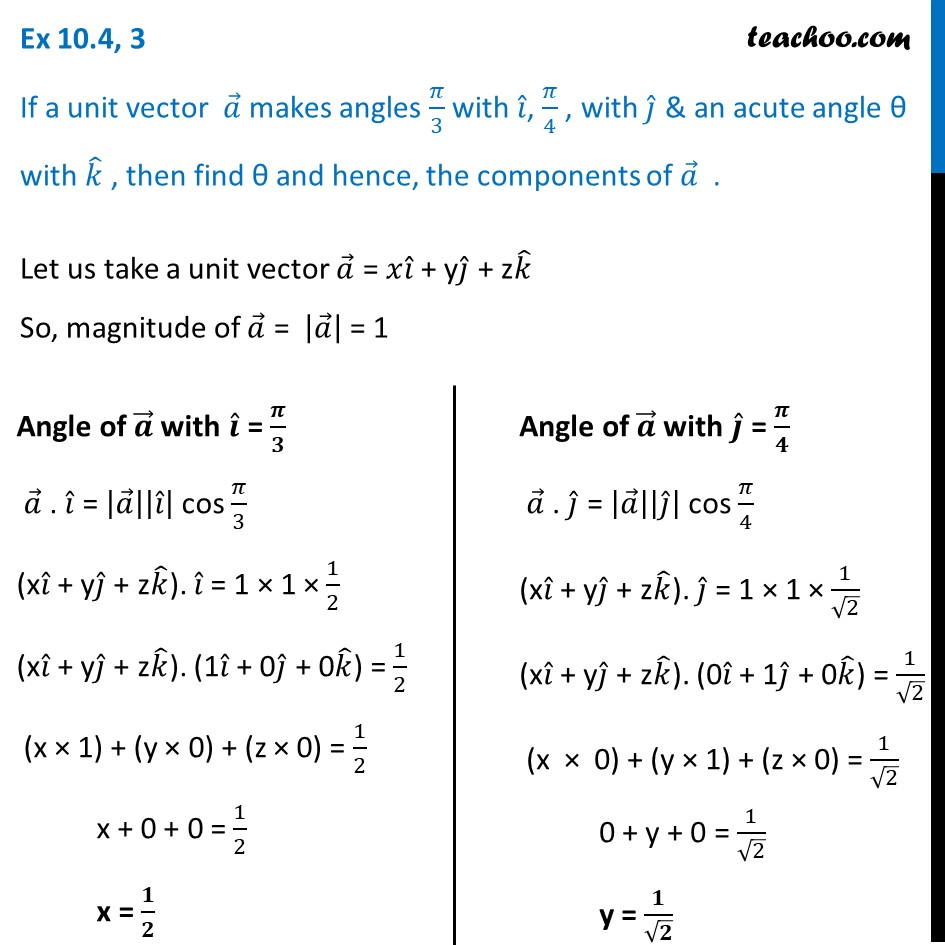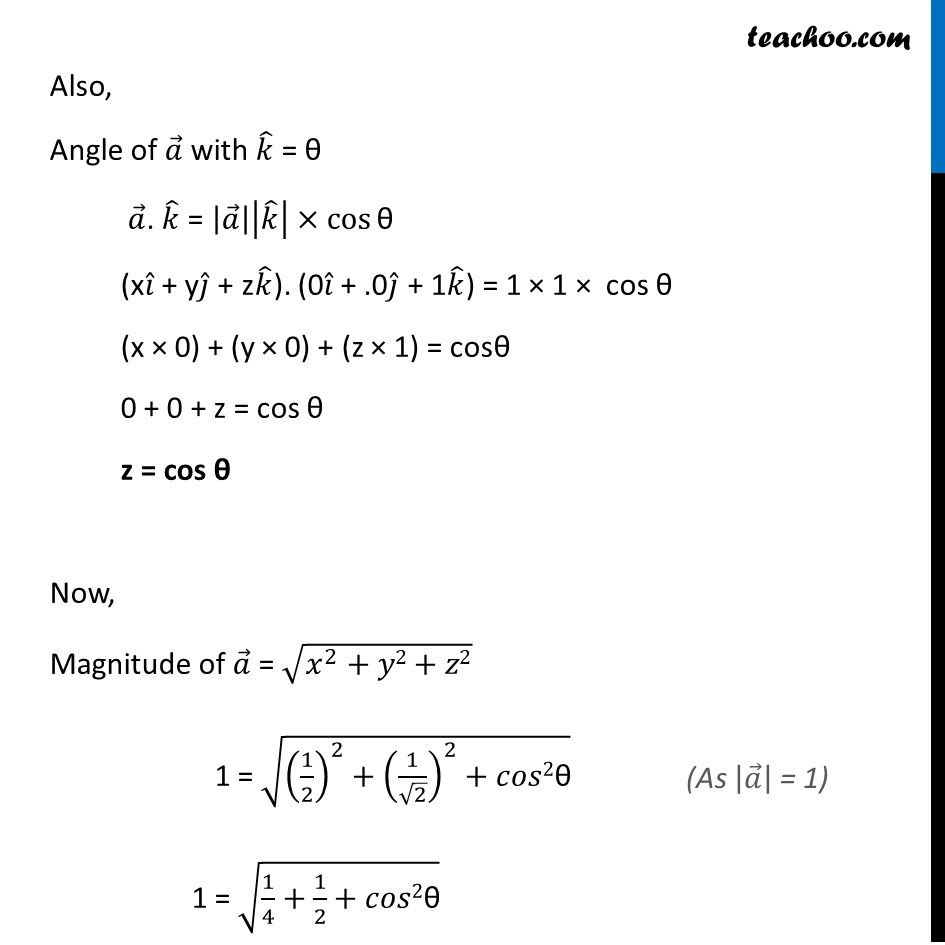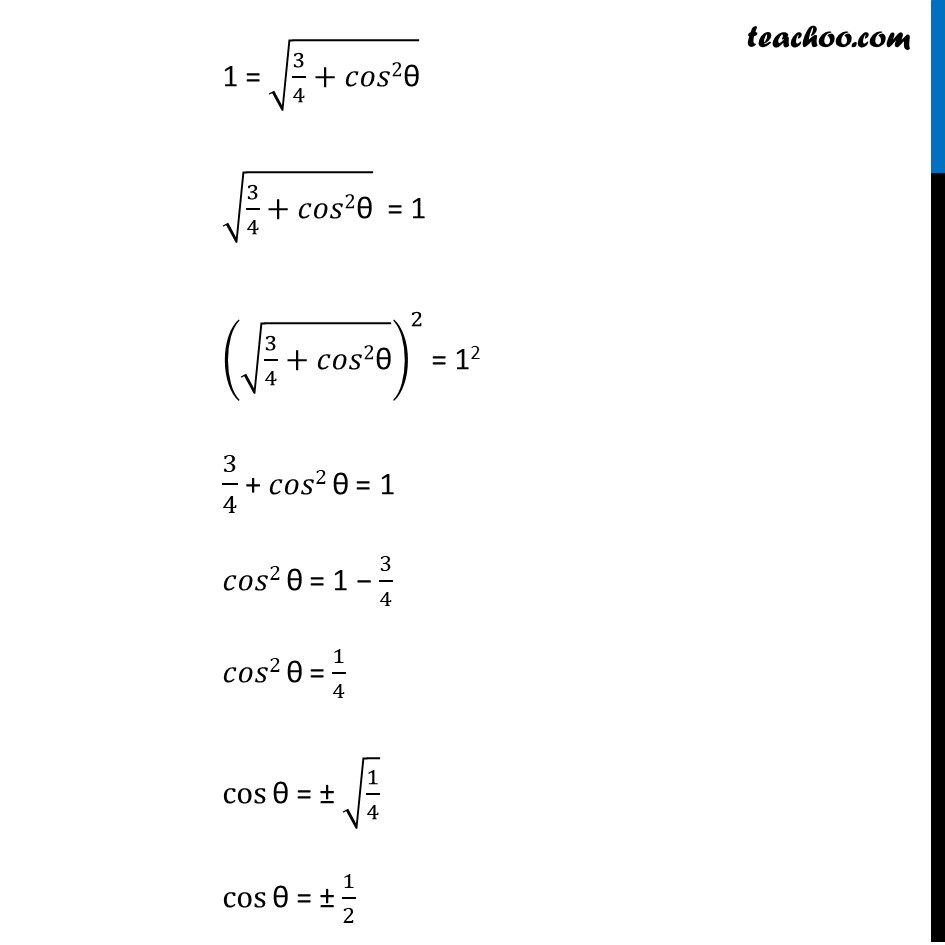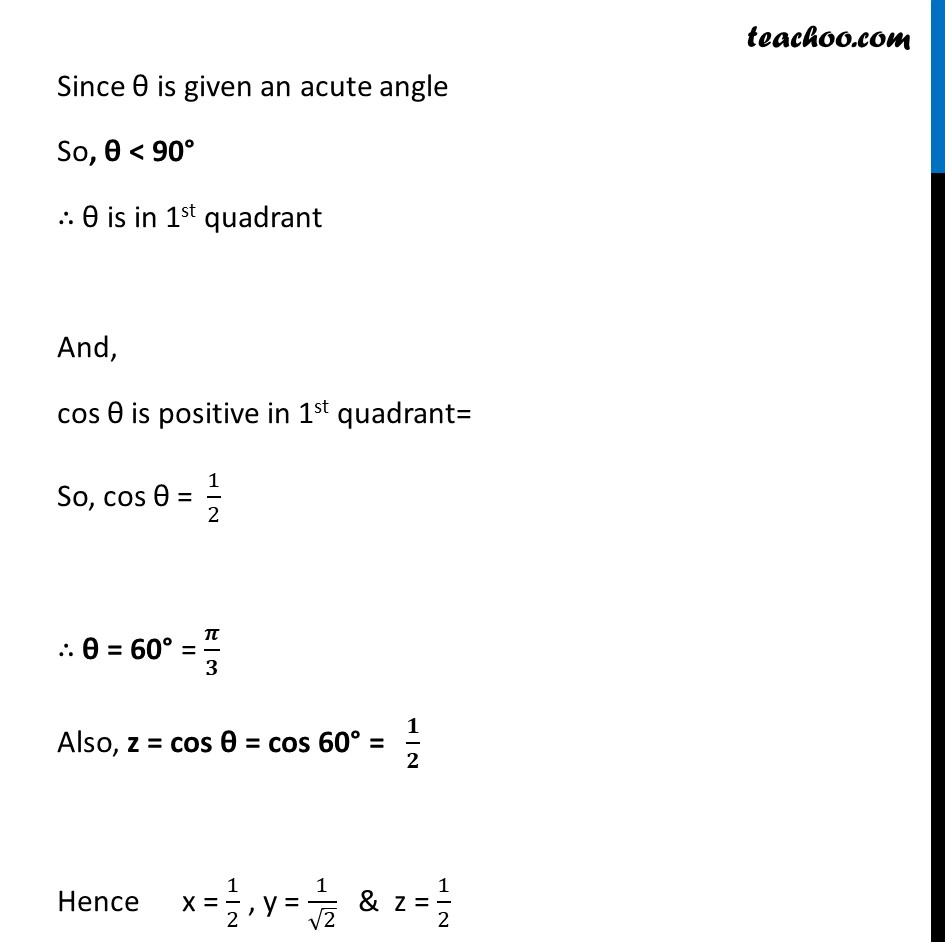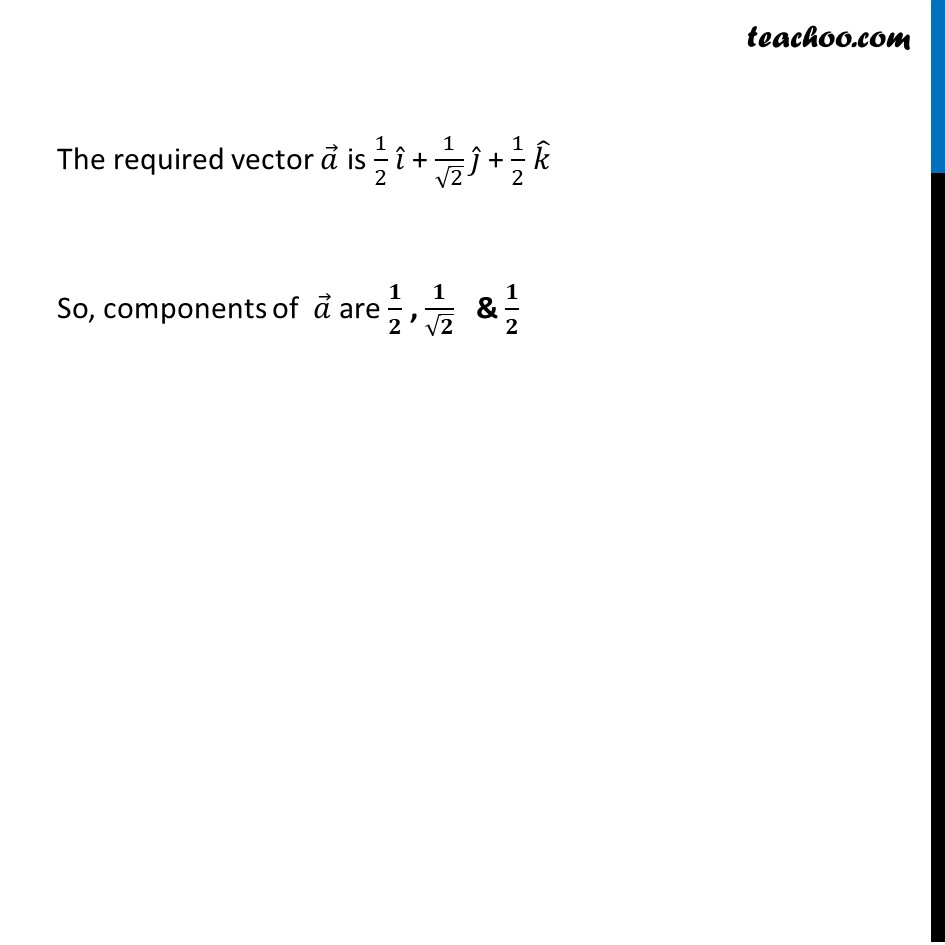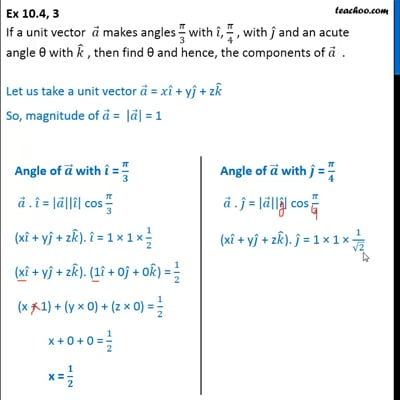This video is only available for Teachoo black users

Introducing your new favourite teacher - Teachoo Black, at only ₹83 per month

### Transcript

Ex 10.4, 3 If a unit vector 𝑎 ⃗ makes angles 𝜋/3 with 𝑖 ̂, 𝜋/4 , with 𝑗 ̂ & an acute angle θ with 𝑘 ̂ , then find θ and hence, the components of 𝑎 ⃗ . Let us take a unit vector 𝑎 ⃗ = 𝑥𝑖 ̂ + y𝑗 ̂ + z𝑘 ̂ So, magnitude of 𝑎 ⃗ = |𝑎 ⃗ | = 1 Angle of 𝒂 ⃗ with 𝒊 ̂ = 𝝅/𝟑 𝑎 ⃗ . 𝑖 ̂ = |𝑎 ⃗ ||𝑖 ̂ | cos 𝜋/3 (x𝑖 ̂ + y𝑗 ̂ + z𝑘 ̂). 𝑖 ̂ = 1 × 1 × 1/2 (x𝑖 ̂ + y𝑗 ̂ + z𝑘 ̂). (1𝑖 ̂ + 0𝑗 ̂ + 0𝑘 ̂) = 1/2 (x × 1) + (y × 0) + (z × 0) = 1/2 x + 0 + 0 = 1/2 x = 𝟏/𝟐 Angle of 𝒂 ⃗ with 𝒋 ̂ = 𝝅/𝟒 𝑎 ⃗ . 𝑗 ̂ = |𝑎 ⃗ ||𝑗 ̂ | cos 𝜋/4 (x𝑖 ̂ + y𝑗 ̂ + z𝑘 ̂). 𝑗 ̂ = 1 × 1 × 1/√2 (x𝑖 ̂ + y𝑗 ̂ + z𝑘 ̂). (0𝑖 ̂ + 1𝑗 ̂ + 0𝑘 ̂) = 1/√2 (x × 0) + (y × 1) + (z × 0) = 1/√2 0 + y + 0 = 1/√2 y = 𝟏/√𝟐 Also, Angle of 𝑎 ⃗ with 𝑘 ̂ = θ 𝑎 ⃗. 𝑘 ̂ = |𝑎 ⃗ ||𝑘 ̂ |×cos⁡"θ" (x𝑖 ̂ + y𝑗 ̂ + z𝑘 ̂). (0𝑖 ̂ + .0𝑗 ̂ + 1𝑘 ̂) = 1 × 1 × cos θ (x × 0) + (y × 0) + (z × 1) = cosθ 0 + 0 + z = cos θ z = cos θ Now, Magnitude of 𝑎 ⃗ = √(𝑥^2+𝑦2+𝑧2) 1 = √((1/2)^2+(1/√2)^2+𝑐𝑜𝑠2"θ" ) 1 = √(1/4+1/2+𝑐𝑜𝑠2"θ" ) 1 = √(3/4+𝑐𝑜𝑠2"θ" ) √(3/4+𝑐𝑜𝑠2"θ" ) = 1 (√(3/4+𝑐𝑜𝑠2"θ" ))^2 = 12 3/4 + 𝑐𝑜𝑠2" θ" = 1 𝑐𝑜𝑠2 "θ" = 1 − 3/4 𝑐𝑜𝑠2" θ" = 1/4 cos⁡"θ" = ± √(1/4) cos⁡"θ" = ± 1/2 Since θ is given an acute angle So, θ < 90° ∴ θ is in 1st quadrant And, cos θ is positive in 1st quadrant= So, cos θ = 1/2 ∴ θ = 60° = 𝝅/𝟑 Also, z = cos θ = cos 60° = 𝟏/𝟐 Hence x = 1/2 , y = 1/√2 & z = 1/2 The required vector 𝑎 ⃗ is 1/2 𝑖 ̂ + 1/√2 𝑗 ̂ + 1/2 𝑘 ̂ So, components of 𝑎 ⃗ are 𝟏/𝟐 , 𝟏/√𝟐 & 𝟏/𝟐